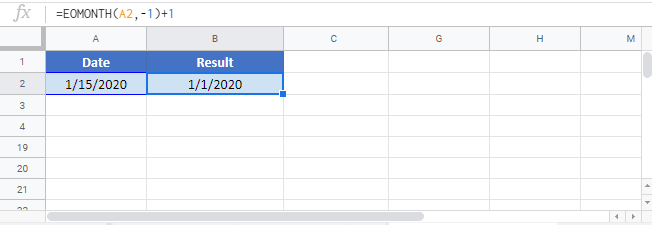# First Day of Month – Formulas in Excel and Google Sheets

This tutorial will teach you how to calculate the first day of the month in Excel and Google Sheets.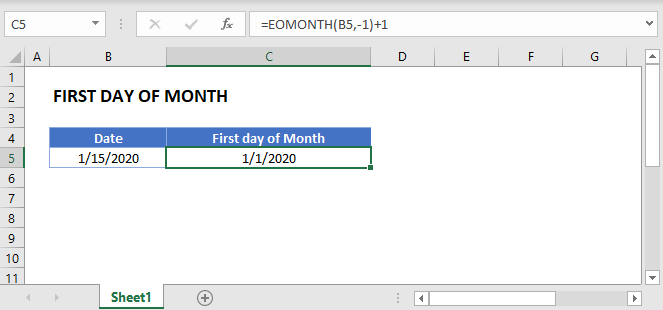## Calculate First Day of the Month

### EOMONTH Function

There’s no Excel function to calculate the first day of the month, but the EOMONTH function will calculate the last day of a month.

To find the first day of a month we will calculate the last day of the previous month and add one day.

Here let’s find the last day of the previous month:

``=EOMONTH(B3,-1)``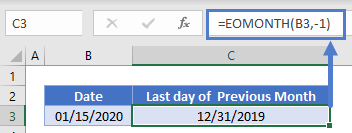Now let’s add one day to the result:

``=EOMONTH(B3,-1)+1``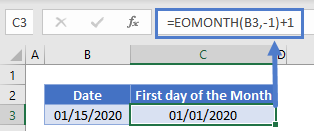### DAY Function

You can also use the DAY Function to find the first day of the month:

``=B3 - DAY(B3)+1``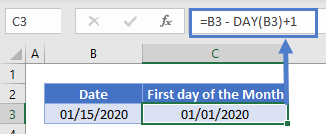Here the DAY Function returns the day number of a date. By subtracting this from the current date (and adding 1) we arrive at the first day of the month.